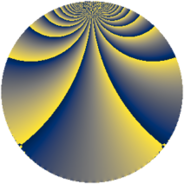# Properties

 Label 684.3.xLevel $684$ Weight $3$ Character orbit 684.x Rep. character $\chi_{684}(7,\cdot)$ Character field $\Q(\zeta_{6})$ Dimension $472$ Sturm bound $360$

# Related objects

## Defining parameters

 Level: $$N$$ $$=$$ $$684 = 2^{2} \cdot 3^{2} \cdot 19$$ Weight: $$k$$ $$=$$ $$3$$ Character orbit: $$[\chi]$$ $$=$$ 684.x (of order $$6$$ and degree $$2$$) Character conductor: $$\operatorname{cond}(\chi)$$ $$=$$ $$684$$ Character field: $$\Q(\zeta_{6})$$ Sturm bound: $$360$$

## Dimensions

The following table gives the dimensions of various subspaces of $$M_{3}(684, [\chi])$$.

Total New Old
Modular forms 488 488 0
Cusp forms 472 472 0
Eisenstein series 16 16 0

## Trace form

 $$472 q - 2 q^{2} - 2 q^{4} + 2 q^{5} + 15 q^{6} - 8 q^{8} + 6 q^{9} + O(q^{10})$$ $$472 q - 2 q^{2} - 2 q^{4} + 2 q^{5} + 15 q^{6} - 8 q^{8} + 6 q^{9} - 10 q^{10} + 5 q^{12} - 4 q^{13} + 9 q^{14} - 2 q^{16} + 20 q^{17} - 22 q^{18} - 55 q^{20} + 16 q^{21} + 9 q^{22} - 43 q^{24} - 1098 q^{25} + 32 q^{26} - 18 q^{28} + 2 q^{29} + 135 q^{30} - 2 q^{32} + 96 q^{33} - q^{34} - 131 q^{36} - 16 q^{37} + 225 q^{38} + 86 q^{40} - 58 q^{41} - 196 q^{42} + 93 q^{44} - 58 q^{45} + 84 q^{46} - 83 q^{48} + 1480 q^{49} + 15 q^{50} - 130 q^{52} - 4 q^{53} + 14 q^{54} - 84 q^{56} + 66 q^{57} - 10 q^{58} + 31 q^{60} + 2 q^{61} - 402 q^{62} + 256 q^{64} - 152 q^{65} - 321 q^{66} + 14 q^{68} + 10 q^{69} + 60 q^{70} + 405 q^{72} + 8 q^{73} - 304 q^{74} + 93 q^{76} - 396 q^{77} - 142 q^{78} + 68 q^{80} - 122 q^{81} - 10 q^{82} + 230 q^{84} + 196 q^{85} - 78 q^{86} + 126 q^{88} + 140 q^{89} - 211 q^{90} + 30 q^{92} - 38 q^{93} + 6 q^{94} - 77 q^{96} - 124 q^{97} + 16 q^{98} + O(q^{100})$$

## Decomposition of $$S_{3}^{\mathrm{new}}(684, [\chi])$$ into newform subspaces

The newforms in this space have not yet been added to the LMFDB.